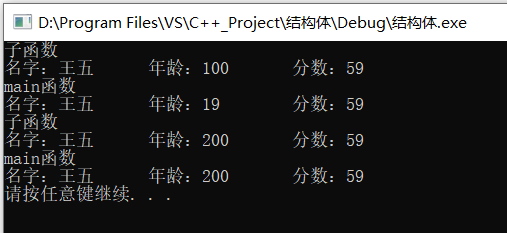• #include<iostream> using namespace std; #include<... *结构体作为函数参数传参 */ //值传递 void printStufdent1(struct Student st3) { cout << "子函数" << endl; st3.age .
#include<iostream>
using namespace std;

#include<string>

//结构体
struct Student {

string name;
int age;
int score;

}st3;

/*
*结构体作为函数参数传参
*/
//值传递
void printStufdent1(struct Student st3) {
cout << "子函数" << endl;
st3.age = 100;

cout << "名字：" << st3.name << "	年龄：" << st3.age << "	分数：" << st3.score << endl;

}
//地址传递
void printStufdent2(struct Student * p) {
p->age = 200;
cout << "子函数" << endl;
cout << "名字：" << p->name << "	年龄：" << p->age << "	分数：" << p->score << endl;

}

int main() {

struct Student st1;
st1.name = "zhangsan";
st1.age = 18;
st1.score = 60;
//cout << "名字" << st1.name << "年龄" << st1.age << "分数" << st1.score<< endl;
struct Student st2={"李四",20,70};
//	cout << "名字" << st2.name << "年龄" << st2.age << "分数" << st2.score<< endl;

st3.name = "王五";
st3.age = 19;
st3.score = 59;

printStufdent1(st3);
cout << "main函数" << endl;
cout << "名字：" << st3.name << "	年龄：" << st3.age << "	分数：" << st3.score << endl;

printStufdent2(&st3);
cout << "main函数" << endl;
cout << "名字：" << st3.name << "	年龄：" << st3.age << "	分数：" << st3.score << endl;

system("pause");
}从结果我们知道结构体作为函数的参数传参有两种形式 
展开全文c++
• 结构体作为函数参数结构体作为函数参数时，参数传递的方式为“传值调用”，即将当前的值复制一份给函数的接口参数。 示例如下： /* * 需求：给定2个学生的信息，包括姓名、学号及5门课的成绩，要求利用函数计算每...
结构体作为函数参数
当结构体作为函数参数时，参数传递的方式为“传值调用”，即将当前的值复制一份给函数的接口参数。 示例如下：
/*
* 需求：给定2个学生的信息，包括姓名、学号及5门课的成绩，要求利用函数计算每个学生的平均成绩
*
*/
#include <stdio.h>
// 定义结构体类型
struct Student
{
char name;
long num;
float ave;
} ;

void calc_average(struct Student s);  // 计算单个学生的平均成绩
void calc_array_average(struct Student s[]);  // 对多个学生计算每个人的平均成绩

int main()
{
int i;
struct Student s = {  //声明并初始化2个学生的数据
{
"ZhangSan", 10001, {60,70,80,90,100}, 0
},
{
"Lisi", 10002, {60, 70, 60, 70, 60}, 0
}
};
// calc_array_average(s); // 计算单个学生的平均成绩，参数为单个结构体，此时输出的结果为0
calc_array_average(s);  // 将结构体数组作为参数，为每个学生计算平均成绩，此时能得到正确的结果
for (i = 0; i < 2; i++) // 输出每个学生的平均成绩
printf("s[%d].ave=%f\n", i, s[i].ave);
return 0;
}

void calc_average(struct Student s)  // 传值调用
{
int i;
float sum = 0;
for (i = 0; i < 5; i++)
s.ave = sum / 5;
}
void calc_array_average(struct Student s[])  // 传引用调用，因为数组名是常量指针，传递的是地址
{
int i, j;
float sum = 0;
for (i = 0; i < 2; i++)
{
for (j = 0,sum = 0; j < 5; j++)
{
}
s[i].ave = sum / 5;
}
return;
}

展开全文• #include &lt;iostream&gt;#include &lt;string&...结构体与函数 结构体作为函数参数、结构体作为函数返回值---------------------------------*/struct time{ int hour; int mi...
#include <iostream>
#include <string>
using namespace std;
/*---------------------------------
18.39.结构体与函数
结构体作为函数参数、结构体作为函数返回值
---------------------------------*/
struct time{
int hour;
int minute;
};
const int perhour=60;
time sum(time t1,time t2);
void show(time t);
int main()
{
time one={8,15};
time two={6,46};
time day=sum(one,two);
show(one);
show(two);
cout<<"两天的时间总计：";
show(day);
time three={2,10};
show(three);
cout<<"三天的时间总计：";
time day2=sum(day,three);
show(day2);
return 0;
}
time sum(time t1,time t2)
{
time total;
total.minute=(t1.minute+t2.minute)%perhour;
total.hour=t1.hour+t2.hour+(t1.minute+t2.minute)/perhour;
}
void show(time t)
{
cout<<"hour:"<<t.hour<<"\tminute:"<<t.minute<<endl;
}

运行结果：

hour:8  minute:15
hour:6  minute:46
两天的时间总计：hour:15 minute:1
hour:2  minute:10
三天的时间总计：hour:17 minute:11
Press any key to continue

展开全文• 结构体作为函数参数 作用：将结构体作为参数向函数中传递 一般来说函数的参数传递方式有两种： 值传递 地址传递 具体可以参照这边文章 https://blog.csdn.net/zhang2039/article/details/108547625一般来说函数的...
结构体作为函数参数
作用：将结构体作为参数向函数中传递 一般来说函数的参数传递方式有两种： 值传递 地址传递 具体可以参照这边文章

https://blog.csdn.net/zhang2039/article/details/108547625一般来说函数的参数传递方式有两种： 值传递 地址传递

结构体作为函数的参数传递使用的就是引用传递，这样做的好处就是它的效率和指针一样高。当结构体很大的时候，避免传递结构体变量很大的值，节省内存，提高效率
案例分析：
#include<iostream>
using namespace std;
#include<string>
struct student {
//成员列表
string name;  //姓名
int age;      //年龄
int score;    //分数
};
//值传递
void printStudent(student stu ) {
stu.age = 18;
cout << " 姓名：" << stu.name << " 年龄： " << stu.age  << " 分数：" << stu.score << endl;
}
//地址传递 void printStudent2(student *stu) {
stu->age = 28;
cout << "子函数中 姓名：" << stu->name << " 年龄： " << stu->age  << " 分数：" << stu->score << endl;
}
int main() {
//定义结构体变量，并初始化。
struct student stu = { "小明",6,100, };

//值传递
printStudent(stu);
cout << "主函数中 姓名：" << stu.name << " 年龄： " << stu.age << " 分数：" << stu.score << endl;
cout << endl;
//地址传递
printStudent2(&stu);
cout << "主函数中 姓名：" << stu.name << " 年龄： " << stu.age  << " 分数：" << stu.score << endl;

system("pause");
return 0;

}

展开全文• #include &lt;iostream&gt;#include &lt;string&...结构体与函数 结构体作为函数参数、结构体指针作为函数返回值---------------------------------*/struct time{ int hour; int ...
• *结构体作为函数参数传参 */ //值传递 void printStufdent1(struct Student st3) { cout << 子函数 << endl; st3.age = 100; cout << 名字： << st3.name << 年龄： << ...
• 结构体作为函数参数 创建一个结构体 struct Student { //成员列表 string name; int age; int score; }; 值传递 //结构体作为函数参数 值传递 void printStu(Student s) { cout << "姓名： " << s...c++
• 结构体作为函数参数 结构体作为函数参数有三种传递方式： 1、传递结构体成员 2、传递结构体本身 3、传递结构体地址 # include<stdio.h> struct Account { char* bankName;//银行账户名称 char* ...c语言 编程语言 指针
• 结构变量是一个标量，它可以用于其他标量可以使用的任何场合，但把结构体作为参数传递给一个函数要注重效率 例如下面这样一个结构体： #define PRODUCT_SIZE 20 typedef struct{ char product[PRODUCT_SIZE]; ...struct
• 一般来讲,我们会把初始化结构体的代码提取出来,封装到init()函数中,而通过将结构体变量作为函数参数来完成初始化,但有的时候比如,结构体变量作为参数,在初始化函数中用malloc动态分配内存,这样的行为不能完成外面的...c语言 指针
• 经过验证，go语言结构体作为函数参数，采用的是值传递。所以对于大型结构体传参，考虑到值传递的性能损耗，最好能采用指针传递。 验证代码： package main import ( "fmt" ) type st struct { id ...
• 1.demo:计算成绩及格的学生个数 #include <...struct Student{ //建立结构体类型struct Student char name; //名字 char id; //学号 int score; //成绩 }temp; int judge_pass(struct St...
• 在利用结构体作为函数参数进行传递时，容易犯的一个错误是将一个野指针传给函数导致错误。 #include #include #include #define MAXSIZE 10 typedef struct { int r[MAXSIZE]; //用于存储要排序的数组，...
• 结构体虽然和数组一样，都可以存储多个数据项，但是在涉及到函数时，结构变量的行为更接近于一个基本的单值变量，也就是说，与数组不同，结构将其数据组合成单个实体或数据对象，该实体被视为一个整体。函数参数为...C
• 在做小项目时遇到一个问题，将main函数结构体作为参数传递时，在函数中修改结构体的值，出了函数后不会修改实参的值，即C语言中将结构体作为参数传递时，并不会改变实参的值！ 原因是当结构体指针传递给函数时，会...指针 c语言
• #define _CRT_SECURE_NO_WARNINGS #include ...//定义一个结构体 typedef struct _Teacher { char name; int age; }Teacher; int printfArr(Teacher *to,int len) { int i = 0; for ( i = 0; ic语言 指针
• struct Books { int a; char b; float c; };shell...